# Quiz 5: Using Equations to Solve Business Problems

Business

Solving for the unknown and proving the solution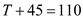The equationindicates addition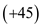. To solve for T , apply the opposite operation, subtraction. Subtract 45 from each side.Thus the solution is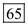. Proof: Substitute 65 for TA(n) formula For example the simple interest formula,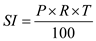, Where interest equals principal times rate times time.

Solving for the unknown and proving the solution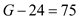The equationindicates subtraction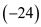. To solve for G , apply the opposite operation, addition. Add 24 from each side.Thus the solution is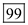. Proof: Substitute 99 for GThere is no answer for this question

There is no answer for this question

There is no answer for this question

There is no answer for this question

There is no answer for this question